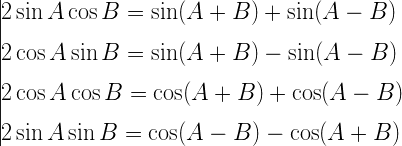Q

# Help me understand! If in a triangle ABC, ÐC =90°, then the maximum value of sinA sinB is

If in a triangle ABC, ÐC =90°, then the maximum value of sinA sinB is

• Option 1)

1/2

• Option 2)

1

• Option 3)

2

• Option 4)

None of these

214 Views

As we learnt

Transformation Formulae -- wherein

These formula are also called A-B formulae.

sinA sinB =        =

==  £

Þ Maximum value of sinA sinB =.

Option 1)

1/2

Option 2)

1

Option 3)

2

Option 4)

None of these

Exams
Articles
Questions